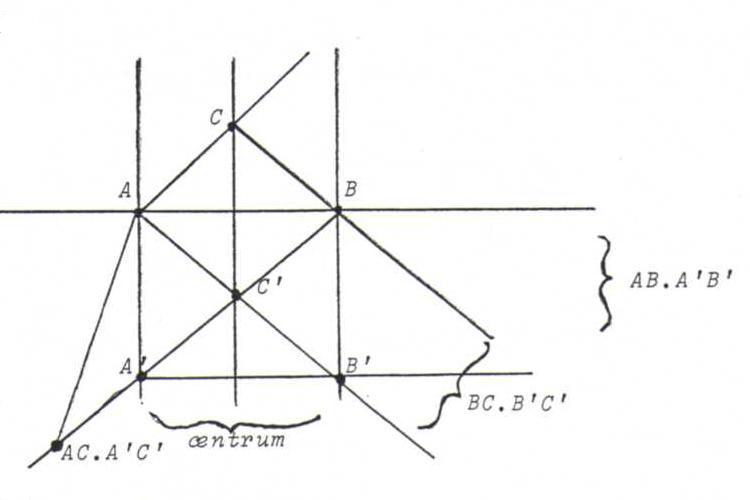### COURSE OF PROJECTIVE GOMETRY

§ 3: answers

O8 Desargues: given two triangles a1b1c1 and a2b2c2, where a1.a2, b1.b2 and c1.c2 are lying on one line (then we call the triangles line perspective); then the connecting lines a1b1-a2b2, a1c1-a2c2 and b1c1-b2c2 concur (we call the triangles correspondingly point perspective).
Notice that the dual theorem of Desargues is the reverse of the theorem of Desargues: together they form the theorem that two triangles are point perspective if and only if they are (correspondingly) line perspective.

Pappus: Given two points L and M, three lines a1,a2,a3 through L, and three lines b1,b2,b3 through M. Then the connecting lines a1b2-a2b1, a1b3-a3b1 and a3b2-a2b3 concur.

O9 Using Desargues: choose two points A1 and B1 on l and two points A2 and B2 on m, so that l.m = A1B1. A2B2 = T.
Make a Desargues configuration with triangles A1B1C1 and A2B2C2 that are point perspective with center T, so that P = A1C1. A2C2.
Then the required line goes through P and B1C1. B2C2.

O10 Using Pappus: draw a line m that doesn't coincide with l and not through A or B. Choose points L1,L2,L3 on l and M1,M2,M3 on m so that A = L1M2. L2M1, B = L2M3. L3M2. Then the line through A and B is the line through B and L1M3. L3M1.

General method: first make a configuration of Desargues or (dual) Pappus, and find therein three points on a line or three lines through a point, whatever the problem requires.
Now make the construction by adding an element of the configuration again and again, as the problem requires, checking each time whether some elements of the configuration are now fixed or that they all can be chosen freely.

O11ABC and A'B'C' are point perspective, but not line perspective.